Pre-digital Calculating, Filing & Weather Forecasting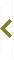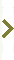# Slide rules and how they were used for calculating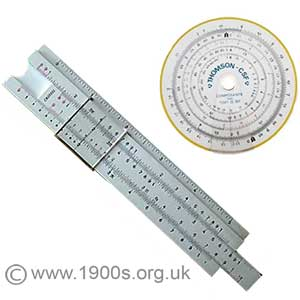The slide rule was a device widely used for making calculations quickly and easily before the development of the electronic calculator. This page describes its basic structure, how it can be used for multiplication and division and its accuracy. It also comments on other functions and variations in structure. Highlights are the recollections from people who used slide rules as a norm.

## Structure of a basic slide rule

The following photo shows a fairly basic slide rule which is the one that I used regularly in the 1960s and 1970s. It is nominally 12 inches long, about 25 cm. It consists of a wide rule, a narrow slider which slides along the central groove and a cursor which is spring-loaded so that it stays in whatever position it is moved to.By sliding the narrow ruler to a suitable position, and reading off from one of the other scales, all sorts of complex calculations can be made quickly and easily - more of which below. For straightforward multiplication and division, only the lower scale on the top fixed rule and the top scale on the slider are used. The other scales are for different calculations which will not be discussed here.

The cursor increases accuracy by enabling markings on the two scales to be lined up more precisely.

My slide rule and most others at the time were made of plastic, but I understand that slide rules made of bone or ivory existed before plastics were developed.

### Slide rules made with plastic and wood

My slide rule in 1952 was wood with a plastic laminated scale surface. I believe that the wood was more dimensionally stable to prevent the slide sticking due to distortion.

## Circular slide rules and 6-inch, half-size slide rules

Slide rules also came - and presumably still come - in different lengths and in circular shapes, but they all have a cursor and work the same way. Circular slide rules are all plastic and very thin.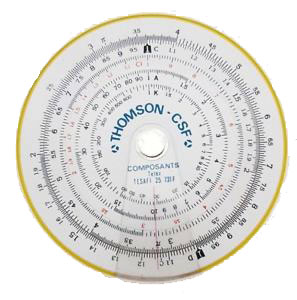A circular slide ruleA short, six inch slide rule

## How slide rules work

You can skip this section if you like and just go on to the next sections on using a slide rule, starting with how to multiply.

Every number has its own unique logarithm, usually just called its log.

Logs are useful because they avoid long multiplication and division of large numbers. The logs of numbers to be multiplied merely have to be added together and the resulting log 'reversed' or anti-logged for the result. Similarly, for division, the logs of the two numbers merely need to be subtracted and anti-logged.

Logs and anti-logs of numbers can be looked up in log tables, but users of slide rules don't have to waste time doing this because the logs of numbers and their logs are already marked on slide rules. Adding and subtracting of the logs are done merely by sliding the scales against each other.

You can see how much quicker this is than consulting the log tables and adding or subtracting them, bearing in mind that when slide rules were popular, there were no electronic calculators.

You will notice from the photos that scales are not linear, i.e. they are scrunched up at the higher end. This is because they are log scales which you can click for more information.

## How to multiply with a slide rule

For multiplication, only the lowest scale on the top part of the slide rule and the upper scale on the slider are used.

Let's take a simple example of multiplying two numbers.

Suppose you want to multiply 2 by 3. Yes, that's so simple that you know the answer anyway, but this is to show the principle.

Move the slider (pale green in the photo) until its 1 is lined up with the first number to be multiplied on the lowest scale on the top part of the slide rule, in this case 2, as shown below in Figure 1.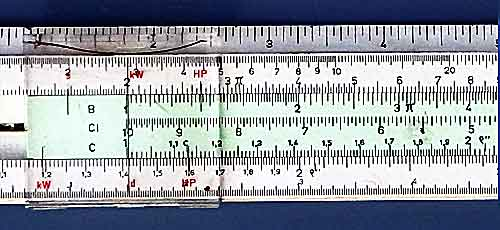Figure 1. Slide rule with the 1 of the sliding scale lined up with the first number to be multiplied on the lowest scale of the top part of the slide rule - in this case 2

Then, with reference to Figure 2, keeping the slider fixed, move the curser to the second number to be multiplied - in this case 3 - and and read off the number it is aligned with on the lowest scale of the top fixed scale - in this case 6. That is the answer. So 2 × 3 = 6Figure 2. Keeping the slider fixed and the cursor moved over the second number to be multiplied - in this case 3 - the answer is shown above it - in this case 6.

By sliding the cursor to various other positions, you can also readily see that other values of 2 times any number can also be read off, for example 2 x 2 = 4 and 2 x 4 = 8 etc.

Using the cursor to mark intermediate positions and moving the 1 of the slider to those positions enables several numbers to be multiplied together in one go.

Where the numbers contain decimals, for example if 3.59 is to be multiplied by 4.62, the cursor becomes more important for accurate reading.

## Decimal points and powers of ten

Slide rules cannot wsho powers of ten or a decimal point. So you need to do a rough answer in your head to see what these should be. For example a multiplication like:

732.8 × 926400

Needs to be treated as:

7 x 102 x 9 x 105

This comes to 63 X 107 which is better expressed as

6.3 x 108

So when the slide rule gives the result as 6.79 you know that the actual result must be

6.79 × 108

This result should be accurate to 3 significant figures which is all that is normally required. In fact it is in some ways unfortunate that electronic calculators give so many significant figures because they are normally meaningless in real situations.

## Division with a slide rule

Division is the reverse of multiplication in that only the same two scales are used and the cursor is placed over the number to be divided and the number that is to divide it is slid along so that the two are lined up. Then the answer is read of from the position of the 1 (or the 10 if the 1 is off the scale).

So 6 ÷ 3 = 2 could be calculated as shown in Figure 2.

## Other slide rule functions

It will not have escaped your notice that there are other scales on the slide rule. These encompass sines, cosines and more advanced functions.

## Accuracy of slide rules

Three significant figures are all that are normally quoted for accuracy with a standard slide rule, but that depends on its length how well it is made. Standard log tables give answers to four figures which which are normally quoted to three significant figures.

### Accuracy of short 6-inch slide rules

I was in the first year that slide rules were allowed in O level exams, and although my maths teacher decried their use as being less accurate than the standard 4 figure log tables, I delighted in getting results that were just as accurate with the six inch slide rule my father bought me. It helped that I was myopic as I could interpolate the small scale quite well and the rule itself was accurate.

Greg Everard

### Accuracy of circular slide rules

I once bought a circular slide rule, but it turned out to have the scale slightly off centre and was very poor even though in theory it should have been accurate to about 6 figures.

Greg Everard